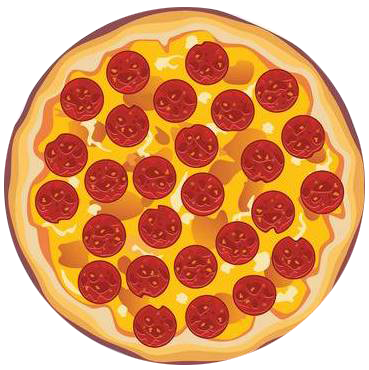IntroductionIntroduction# Fractions

To help us better understand fractions, we like to imagine them as pizzas or legos.

Pick whichever you vibe with more:

INTRO
A fraction tells us how many parts of a certain size there are. Every fraction has a numerator and a denominator.
The numerator is the top number in a fraction and tells us how many equal parts there are.
The denominator is the bottom number and tells us the size and the total number of equal parts needed to make a whole.In the fraction above, the numerator is and the denominator is . So, there is one-fourth slice of pizza or of slices needed to make a whole pizza.
Check out our
Calculator
or explore our
Lesson
and
Practice

CALCULATOR

KEY STEPS

## How to Write Fractions

### Step 1. Identify the denominator.

The denominator represents the size and total number of equal parts needed to make a whole.

### Step 2. Identify the numerator.

The numerator represents how many equal parts there are.

### Step 3. Place the numerator on top of the denominator.

LESSON
Writing Fractions

## Writing Fractions

A fraction tells us how many parts of a certain size there are. The parts can be portions of a single item or portions of a group.There are two-third slices of pizza or of slices needed to make a whole pizza.
The numerator is the top number in a fraction and tells us how many equal parts there are.
The denominator is the bottom number and tells us the size and the total number of equal parts needed to make a whole.
As the size of the denominator increases, the size of the parts decreases.✨ Drag ✨

### Bigger Denominator

Since the number of parts needed to make a whole will always be nonzero, the denominator can never be 0.

### Types of Fractions

 Type Rule Example Proper Fraction top bottom Improper Fraction top bottom Mixed Number whole number fraction
We're just covering proper fractions in this lesson. Check out our lesson on !
Now that we know our basics, let’s check out some examples of how to write fractions!
PRACTICE
Writing Fractions

## Practice: Writing Fractions

Question 1 of 6: What is the fraction represented by this image?### Step 1. Identify the denominator.

The denominator represents how many equal parts a whole is divided into.
How many total slices make up a whole pizza?

CONCLUSION
Incredible job, look at you go! Thanks for checking out this lesson ☺️🙏. Where to next?Leave Feedback